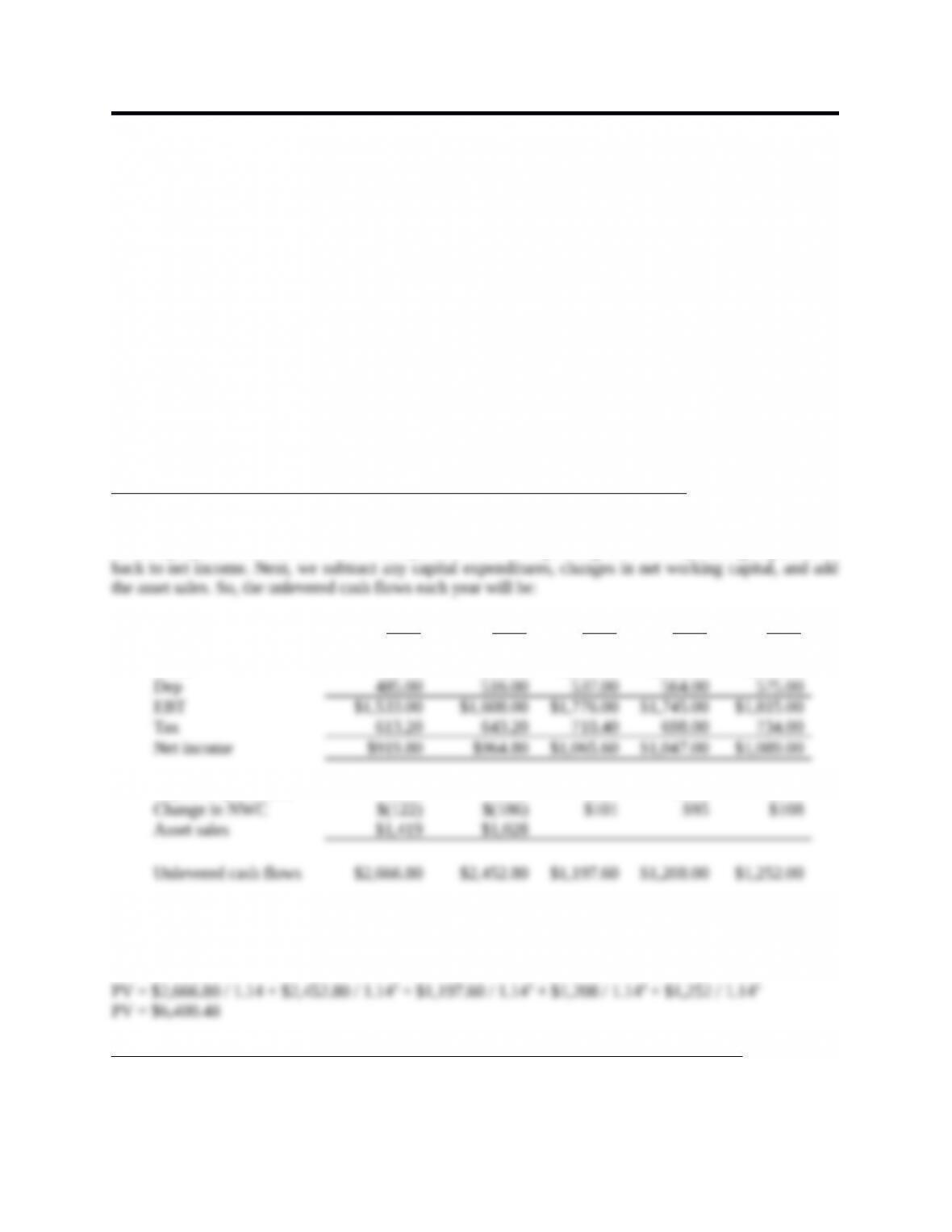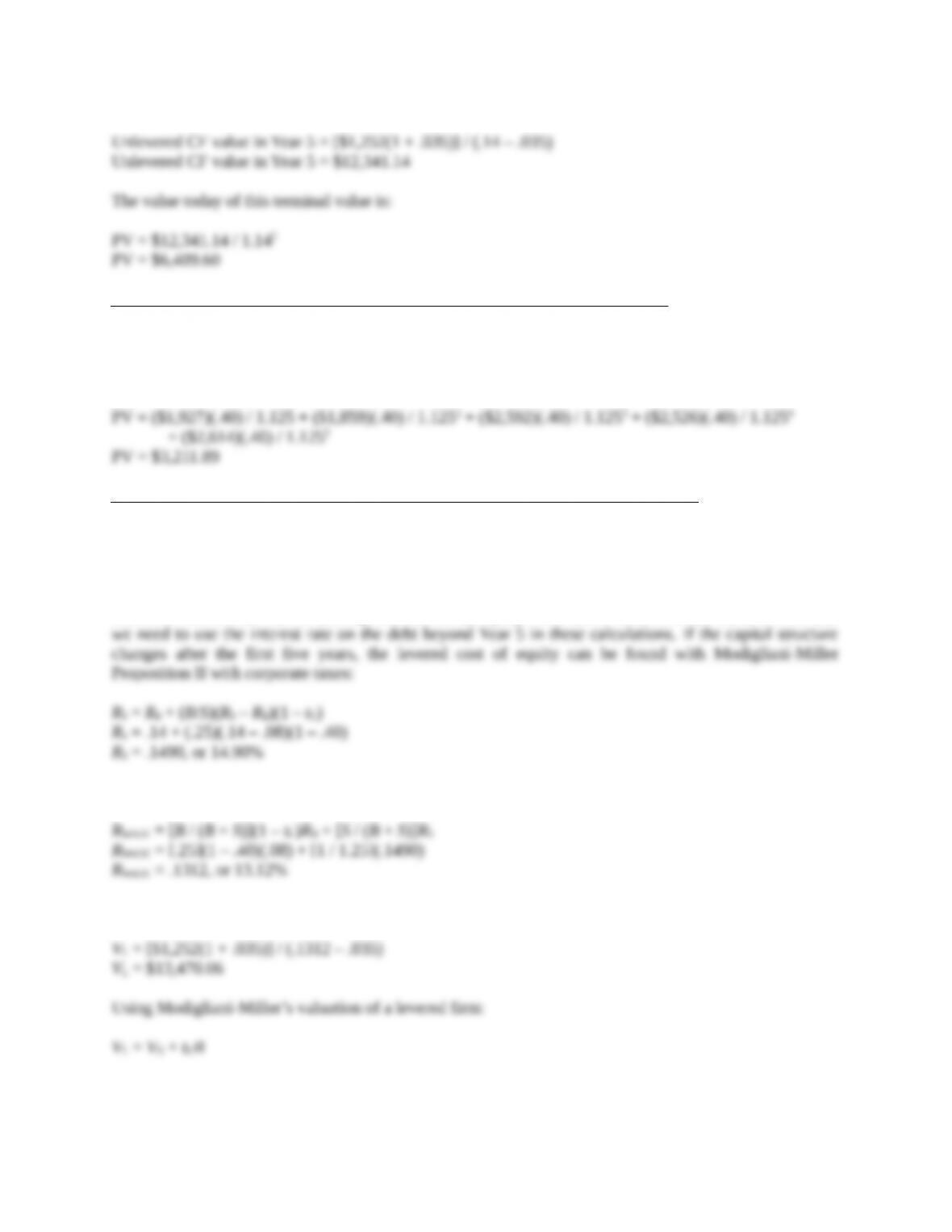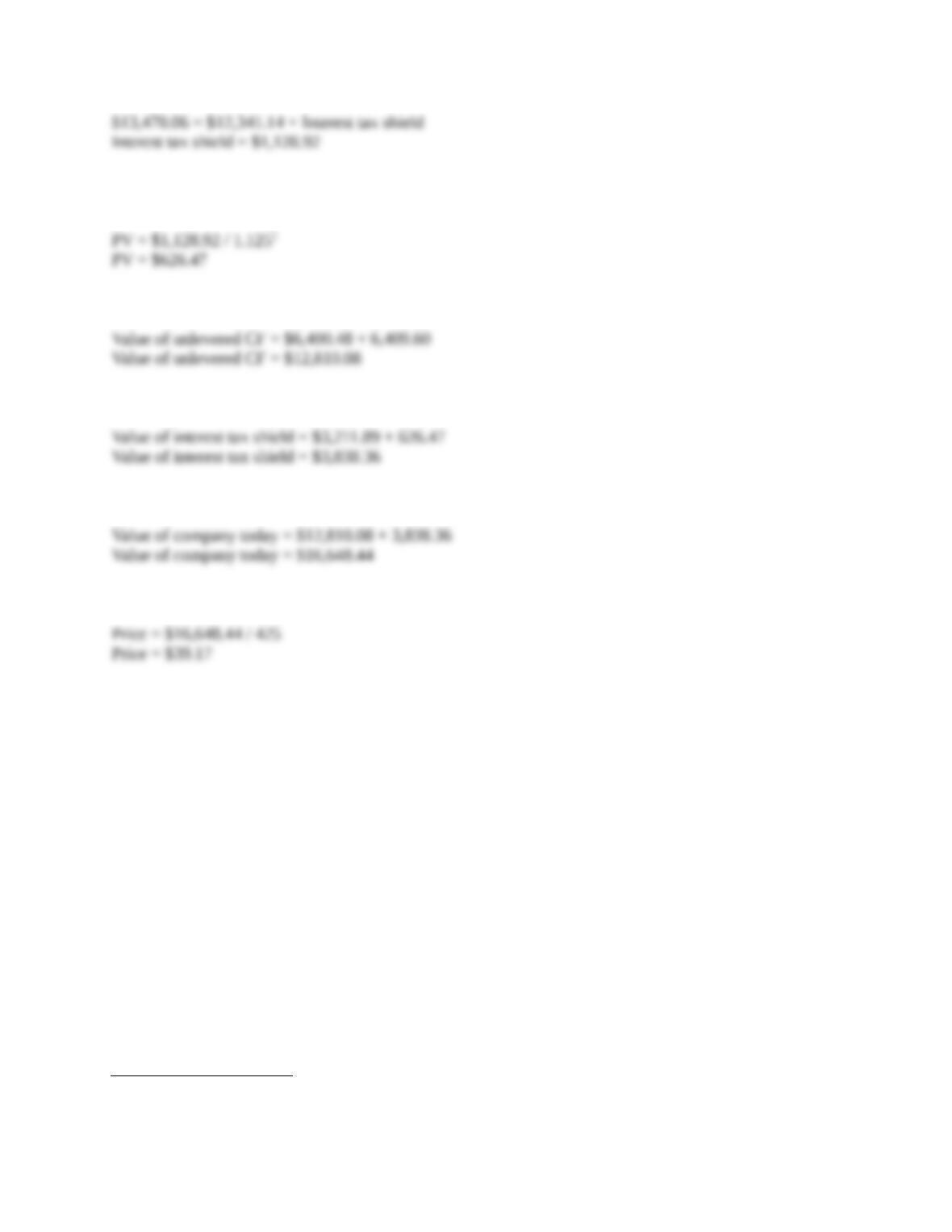Type
Quiz
Book Title
Corporate Finance 11th Edition
ISBN 13
978-1259709685

### 978-1259709685 Chapter 18 Case

December 23, 2019CHAPTER 18 CASE C-1
CHAPTER 18
PRODUCTS, INC.
In this leveraged buyout, the debt level of the company changes through time. Since the debt level
changes through time, the APV method is appropriate for evaluating the LBO. The steps we must
undertake are:
Step 1: Calculating the present value of unlevered cash flows for the first five years.
Step 2: Calculating the present value of the unlevered cash flows beyond the first five years.
Step 3: Calculating the present value of interest tax shields for the first five years.
Step 4: Calculating the present value of interest tax shields beyond the first five years.
Step 1: Calculating the present value of unlevered cash flows for the first five years.
The income statement presented does not include interest, so it is the projected unlevered cash flows of
the company. To find the cash flows each year, we find the operating cash flow by adding depreciation
2015 2016 2017 2018 2019
Sales \$2,749.00 \$3,083.00 \$3,322.00 \$3,400.00 \$3,539.00
Costs 731.00 959.00 1,009.00 1,091.00 1,149.00
Capital expenditures \$279 \$242 \$304 \$308 \$304
Since these are unlevered cash flows, we need to discount at the unlevered cost of equity. Because the
company currently has no debt, the required return on assets is equal to the cost of equity. So, using this
discount rate, we find the present value of the unlevered cash flows for the next five years will be:
Step 2: Calculating the present value of the unlevered cash flows beyond the first five years.
The assumption given is that the cash flows will grow at 3.5 percent into perpetuity. Again, we discount
these cash flows at the unlevered return on equity. So, the value of these cash flows in Year 5 will be:CHAPTER 18 CASE C-2
Step 3: Calculating the present value of interest tax shields for the first five years.
The interest tax shield each year is the interest paid times the tax rate. To find the present value of the
interest tax shield, we need to discount these at the pretax cost of debt, so the present value of the interest
tax shield for the first five years is:
Step 4: Calculating the present value of interest tax shields beyond the first five years.
Finally, we must calculate the value of tax shields associated with debt used to finance the operations of
the company after the first five years. The assumption given in the case is that debt will be reduced and
maintained at 25 percent of the value of the firm from that date forward. Under this assumption it is
appropriate to use the WACC method to calculate a terminal value for the firm at the target capital
structure. This in turn can be decomposed into an all-equity value and a value from tax shields. Note that
Now, we can calculate the WACC for the company beyond Year 5. The WACC at this point will be:
We can use the WACC to calculate the terminal value of the levered company, which will be:
we can value the interest tax shield as:CHAPTER 18 CASE C-3
This is the value of the interest tax shield beyond Year 5. Discounting this at the cost of debt over the first
five years, we find the value today is:1
We have valued all future cash flows of the company. The value of the unlevered cash flows today is:
And the value of the interest tax shield today is:
So, the total value of the company today is:
So, the most the group should offer per share is:
1 A good argument can be made that since post-2019 debt levels are proportional to firm value, the tax
shields are as risky as the firm and should be discounted at the rate R0.